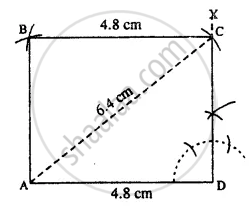# Construct a Rectangle Abcd; If : Ad = 4.8 Cm and Diagonal Ac = 6.4 Cm. - Mathematics

Sum

Construct a rectangle ABCD; if :

AD = 4.8 cm and diagonal AC = 6.4 cm.

#### SolutionSteps :

1. Draw AD = 4.8 cm.
2. At D, draw ∠XDA = 90°.
3. From A, draw an arc of radius 6-4 cm which meets DX at C.
4. From A, draw an arc of radius equal to DC.
5. From C, draw an arc of radius 4.8 cm which meets the first arc at B.
6. Join AB and CB. Thus ABCD is the required rectangle.
Concept: Construction of a Rectangle When Its Length and Breadth Are Given.
Is there an error in this question or solution?

#### APPEARS IN

Selina Concise Mathematics Class 8 ICSE
Chapter 18 Constructions
Exercise 18 (D) | Q 3.4 | Page 211